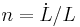User:Issa Rice

test >:(

Questions

• How do we measure growth? How do we know what's growing?
• How is GDP calculated?
• What choices/judgment calls are made in the calculation?
• What's up with all these price indices/PPP formulas/aggregation methods?
• Validation
• Going out and measuring things (surveys? taxation? some old dutch guy at a port kept import records?)
• Can we trust these GDP calculations?

Growth models

Model name Endogenous variables Exogenous variables Constants Year introduced
Harrod–Domar 1939
Solow–Swan 1956
AK
Leontief input–output
Feldman–Mahalanobis
Becker–Barro 1981, 1989
Ramsey–Cass–Koopmans
Romer 1990?
Schumpeterian
Product-variety
Semi-endogenous (Jones)
Production function name Models that use this production function
Cobb–Douglas Solow–Swan
Leontief

Probably for each model, a table like this:

Name Variable Unit Rival input? Endogenous? Notes
Output Y Yes
Physical capital (capital stock) K Yes In Solow–Swan Physical capital includes things like machines, computers, buildings, etc.
Labor L Yes Exogenous in Solow–Swan
Technology (knowledge) A, T No Not in Solow–Swan
Consumption C
Investment I
Amount saved S
Growth of X$g_X = \dot{X}/X = \frac{\frac{\partial X}{\partial t}}{X}$
Population growth$n = \dot L / L$
Depreciation (rate?) δ, d, D
Capital per worker k = K/L In Solow–Swan
Fraction saved s
Output per worker y = Y/L Yes
Time t
Production function F
α